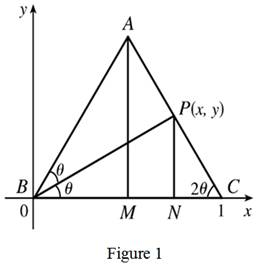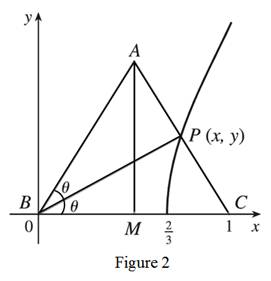# The position of the point P in the given process and to check whether P have a limiting position.### Single Variable Calculus: Concepts...

4th Edition
James Stewart
Publisher: Cengage Learning
ISBN: 9781337687805### Single Variable Calculus: Concepts...

4th Edition
James Stewart
Publisher: Cengage Learning
ISBN: 9781337687805

#### Solutions

Chapter 2, Problem 10P

(a)

To determine

## To find: The position of the point P in the given process and to check whether P have a limiting position.

Expert Solution

The limiting position is 23.

### Explanation of Solution

Graph:Calculation:

Let the coordinate system and drop a perpendicular from P.

From the triangle ΔNCP the angle NCP that tan(2θ)=y1x,

And from the triangle ΔNBP the angle NBP that tan(θ)=yx.

By using double-integral formula for tangents,

y1x=tan(2θ)b±b24ac2ay1x=2tanθ1tan2θy1x=2(yx)1(yx)2y1x=2yx1y2x2

Simplifying above equation

y1x=2yxx2y2x2y1x=2yx×x2x2y2y1x=2xyx2y211x=2xx2y2

Applying cross multiplication

1(x2y2)=2x(1x)x2y2=2x2x2y2=3x22x

y2=x(3x2) (1)

As the altitude AM decreases in length, the point P will approach the x-axis, means y approaches to 0. So the limiting location of P exists and it must be one of the root of the equation. The point P can never be to the left of the altitude AM, so here x not equal to zero, so it must be 3x2=0 implies x=23.

Thus, the limiting position is 23.

(b)

To determine

### To find: The equation of the curve and sketch the path traced out by P.

Expert Solution

The P only traces out the part of the curve with 0y<1.

### Explanation of Solution

Graph:Calculation:

Take the equation (1) from the part (a) y2=x(3x2) is the equation of the curve traced out by P.

As |AM| approach infinity, 2θ approach π2,θ approach π4, x approach 1,

tanθ=yx, y approach 1.

Thus, P only traces out the part of the curve with 0y<1.

### Have a homework question?

Subscribe to bartleby learn! Ask subject matter experts 30 homework questions each month. Plus, you’ll have access to millions of step-by-step textbook answers!